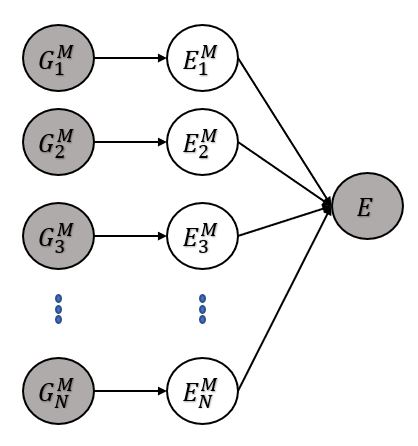# Stacking different BayesianNN

Hello everyone,

I am new in usign pyro and working with the bayesian framework and I wanted to have an opinion on more expert users.

I want to do stacking of BayesianNN as in the figure:Here the labels represents:

G: the features of my data, which are divided in M groups
M: the number of different BayesianNN that will be trained with the M features
E^M: the output value of the M neural network which is a latent variable
E: the observable value that I want to predict, that is the sum of all the E^M_N outputs

This task has already been coded in pytorch with normal NN and the loss is calculated doing back propagation according to the RMSE of the real E and the predicted E.

Do you think is it possible to do something similar with pyro and BNN? Do you know any tutorials that treats a similar case?

Thank you